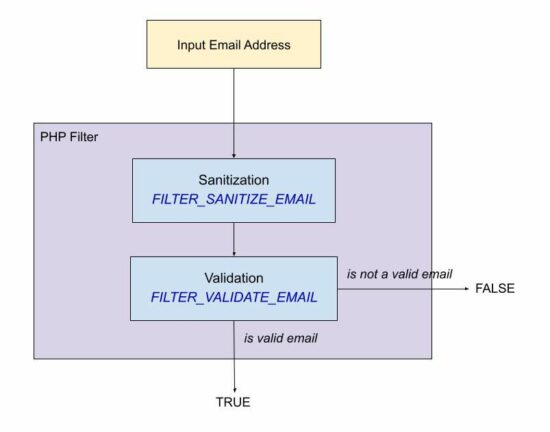# PHP Validate Email using filter_validate_email and regex

Email validation in PHP can be done in different ways. In general, a website will have client-side validation.

I prefer both client and server-side validation. It is applicable not only for emails but also to all inputs received from the end users. It will make the website more robust.

Validating email or other user inputs is a basic precautionary step before processing.

In this article, we will see how to validate email on the server-side. PHP provides various alternates to validate email with its built-in constants and functions. Some of them are listed below.

## Ways to validate email in PHP

1. Using FILTER_VALIDATE_EMAIL.
2. Using pattern matching with regular expression.
3. By validating the domain name from the email address.

The following quick example uses PHP filter FILTER_VALIDATE_EMAIL. It is the best method of validating email in PHP.

## Quick example

``````<?php
\$email = 'test@example.com';
isValidEmail(\$email);

// using FILTER_VALIDATE_EMAIL - this is the best option to use in PHP
function isValidEmail(\$email)
{
return filter_var(\$email, FILTER_VALIDATE_EMAIL) !== false;
}
?>
``````Let us see other methods to validate email using PHP script.

## Using FILTER_SANITIZE_EMAIL for input sanitization

The FILTER_SANITIZE_EMAIL is used to clean the email data submitted by the user.

In this example, the \$email is hard coded with an example email address. You can supply email input from the form data posted to PHP using GET or POST methods.

It adds a prior step to sanitize the email data before validating its format. For validating the format of the sanitized email data, it uses FILTER_VALIDATE_EMAIL.

filter-sanitize-email.php

``````<?php
// this script explains the difference between FILTER_SANITIZE_EMAIL and FILTER_VALIDATE_EMAIL

// validation is to test if an email is in valid email format and FILTER_VALIDATE_EMAIL should be used.
// sanitization is to clean an user input before using it in the program and FILTER_SANITIZE_EMAIL should be used.
\$email = "test@example.com";
\$cleanEmail = filter_var(\$email, FILTER_SANITIZE_EMAIL);

// after sanitization use the email and check for valid email or not
if (filter_var(\$cleanEmail, FILTER_VALIDATE_EMAIL)) {
// the email is valid and use it
}
?>
``````

## Using pattern matching with regular expression

If you are expecting a regex pattern to validate the email format, this example code will help.

This code has a regex pattern in a variable and is used to validate email with PHP preg_match().

In PHP, the preg_match() is for pattern matching with a given subject that is an email address here.

This code has the validateWithRegex() function to process the PHP email validation. It applies converts the input email string to lower case and trims before applying preg_match().

Then, it returns a boolean true if the match is found for the email regex pattern.

email-regex.php

``````<?php
validateWithRegex(\$email);

// Using regular expression (regex). If for some reason you want to validate email via a regex use this
// function. The best way to validate is via FILTER_VALIDATE_EMAIL only.
function validateWithRegex(\$email)
{
\$email = trim(strtolower(\$email));

// the regex I have used is from PHP version 8.1.7 which is used in php_filter_validate_email
// reference: https://github.com/php/php-src/blob/PHP-8.1.7/ext/filter/logical_filters.c#L682
\$emailRegex = '/^(?!(?:(?:\\x22?\\x5C[\\x00-\\x7E]\\x22?)|(?:\\x22?[^\\x5C\\x22]\\x22?)){255,})(?!(?:(?:\\x22?\\x5C[\\x00-\\x7E]\\x22?)|(?:\\x22?[^\\x5C\\x22]\\x22?)){65,}@)(?:(?:[\\x21\\x23-\\x27\\x2A\\x2B\\x2D\\x2F-\\x39\\x3D\\x3F\\x5E-\\x7E\\pL\\pN]+)|(?:\\x22(?:[\\x01-\\x08\\x0B\\x0C\\x0E-\\x1F\\x21\\x23-\\x5B\\x5D-\\x7F\\pL\\pN]|(?:\\x5C[\\x00-\\x7F]))*\\x22))(?:\\.(?:(?:[\\x21\\x23-\\x27\\x2A\\x2B\\x2D\\x2F-\\x39\\x3D\\x3F\\x5E-\\x7E\\pL\\pN]+)|(?:\\x22(?:[\\x01-\\x08\\x0B\\x0C\\x0E-\\x1F\\x21\\x23-\\x5B\\x5D-\\x7F\\pL\\pN]|(?:\\x5C[\\x00-\\x7F]))*\\x22)))*@(?:(?:(?!.*[^.]{64,})(?:(?:(?:xn--)?[a-z0-9]+(?:-+[a-z0-9]+)*\\.){1,126}){1,}(?:(?:[a-z][a-z0-9]*)|(?:(?:xn--)[a-z0-9]+))(?:-+[a-z0-9]+)*)|(?:\\[(?:(?:IPv6:(?:(?:[a-f0-9]{1,4}(?::[a-f0-9]{1,4}){7})|(?:(?!(?:.*[a-f0-9][:\\]]){7,})(?:[a-f0-9]{1,4}(?::[a-f0-9]{1,4}){0,5})?::(?:[a-f0-9]{1,4}(?::[a-f0-9]{1,4}){0,5})?)))|(?:(?:IPv6:(?:(?:[a-f0-9]{1,4}(?::[a-f0-9]{1,4}){5}:)|(?:(?!(?:.*[a-f0-9]:){5,})(?:[a-f0-9]{1,4}(?::[a-f0-9]{1,4}){0,3})?::(?:[a-f0-9]{1,4}(?::[a-f0-9]{1,4}){0,3}:)?)))?(?:(?:25[0-5])|(?:2[0-4][0-9])|(?:1[0-9]{2})|(?:[1-9]?[0-9]))(?:\\.(?:(?:25[0-5])|(?:2[0-4][0-9])|(?:1[0-9]{2})|(?:[1-9]?[0-9]))){3}))\\]))\$/iDu';
if (preg_match(\$emailRegex, \$email) === 1) {
return true;
} else {
return false;
}
}
?>
``````

## By validating the domain name from the email string

This method is a very simple one for validating email. It validates email by ensuring its host DNS validness.

It uses the PHP checkdnsrr() function to validate DNS by a hostname or the site IP address.

This function returns a boolean true if the host has any DNS records found. And thereby, the email in that host can be considered in a valid format.

It follows the below list of steps.

1. It extracts the domain name from the email.
2. It extracts the username prefixed before the @ symbol.
3. It ensures that the username and domain name are not empty.
4. It checks if the DNS details are not empty about the host extracted from the input email.

domain-validate-email.php

``````<?php

// simplest custom email validation using email's domain validation
function validateEmail(\$email)
{
\$isEmailValid = FALSE;

if (! empty(\$email)) {
\$domain = ltrim(stristr(\$email, '@'), '@') . '.';
\$user = stristr(\$email, '@', TRUE);

// validate email's domain using DNS
if (! empty(\$user) && ! empty(\$domain) && checkdnsrr(\$domain)) {
\$isEmailValid = TRUE;
}
}
return \$isEmailValid;
}
?>
``````